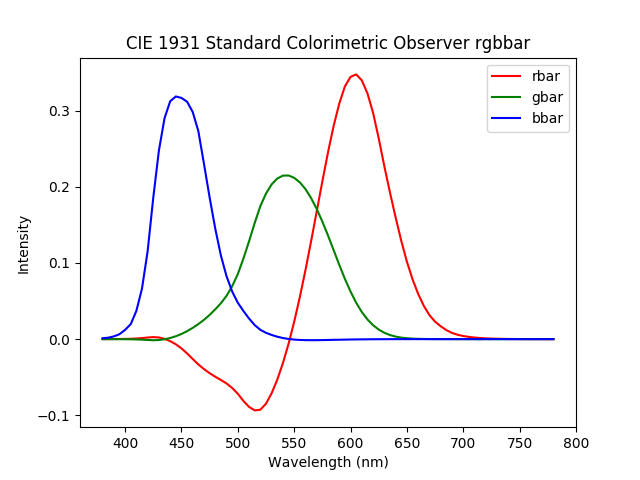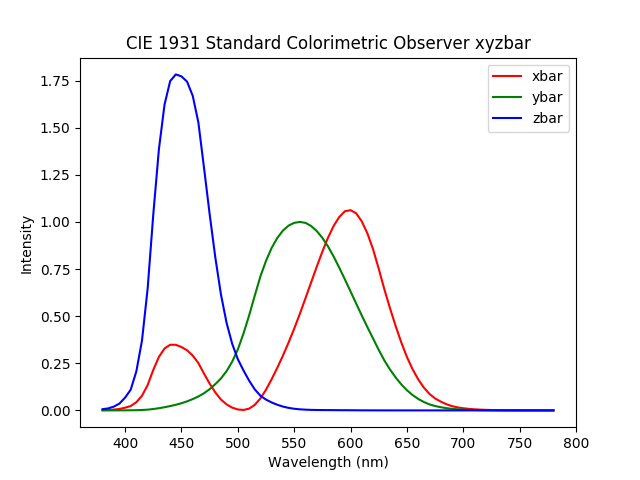# Color fundamentals

Institute for Environmental and Spatial Analysis...University of North Georgia

## 1   Color spectrum

In 1666, Sir Isaac Newton discovered that a beam of sunlight consists of continuous spectrum of colors from violet to red.

## 2   Principal sensing categories in the human eye

• Red: About 65% of cones in the human eye are sensitive to red light.
• Green: 33% are sensitive to green light.
• Blue: 2% are sensitive to blue light, but most sensitive.

## 3   Primary and secondary colors of light

The primary colors of light are defined following these characteristics of the human eye:

• Red (R) produces red light.
• Green (G) produces green light.
• Blue (B) produces blue light.

The secondary colors of light can be produced by adding the primary colors:

• Magenta: R + B
• Cyan: G + B
• Yellow: R + G

R + G + B produces white.

Light sources use this “additive” color system.

## 4   Primary and secondary colors of pigments

The primary colors of pigments are

• Cyan (C) absorbs red light, and reflects green and blue lights.
• Magenta (M) absorbs green light, and reflects red and blue lights.
• Yellow (Y) absorbs blue light, and reflects red and green lights.

The secondary colors of pigments can be produced by subtracting the primary colors:

• Red: M (absorbs green) + Y (absorbs blue)
• Green: C (absorbs red) + Y (absorbs blue)
• Blue: M (absorbs green) + C (absorbs red)

C + M + Y produces black.

Reflective colors (e.g., paints) use this “subtractive” color system.

## 5   Hue, saturation, and brightness

Hue is the dominant color perceived by the human eye.

Saturation refers to the relative purity of a hue or how much the hue is diluted by white light. Pure colors are fully saturated (red vs. pink).

Brightness is the chromatic notion of intensity.

## 6   Chromaticity and tristimulus

Chromaticity refers to the quality of color independent of brightness. That is, hue and saturation considered together in chromaticity.

A color can be described by its chromaticity and brightness.

The amounts of red, green, and blue to reproduce any color are referred to as the tristimulus.

## 7   Commission Internationale de l’Eclairage (CIE) standards

In the 1920s, W. David Wright and John Guild’s experiments

• CIE 1931 standard colorimetric observer
• 2° field of view
• Still widely used
• CIE 1964 standard colorimetric observer
• 10° field of view

## 8   CIE 1931 RGB color space

Additive color space based on the CIE 1931 RGB color model defined by the red, green, and blue additive primaries.

### 8.1   CIE 1931 $\overline{rgb}$ color-matching functions

Data from a color-matching experiment$\int\bar{r}(\lambda)\,d\lambda=\int\bar{g}(\lambda)\,d\lambda=\int\bar{b}(\lambda)\,d\lambda$

These wavelengths were chosen because they were easily reproducible using a mercury vapor discharge at that time:

• Red: 700 nm; Difficult to reproduce, but it was chosen because the perception of color is rather unchanging at this wavelength and insensitive to small errors.
• Green: 546.1 nm
• Blue: 435.8 nm

Notice negative $\bar{r}$ and very slightly negative $\bar{g}$ and $\bar{b}$. Split-screen two halves of a colored disk:

• Left half: Reference color at each wavelength
• Right half: RGB-adjustable using monochromatic primaries

In some cases, the left half had to be adjusted to match the two halves.

### 8.2   CIE 1931 rgb chromaticity coordinates

Rescale $\bar{r}$, $\bar{g}$, and $\bar{b}$ for the same wavelength: \begin{align*} r(\lambda)&=\frac{\bar{r}(\lambda)}{\bar{r}(\lambda)+\bar{g}(\lambda)+\bar{b}(\lambda)},\\ g(\lambda)&=\frac{\bar{g}(\lambda)}{\bar{r}(\lambda)+\bar{g}(\lambda)+\bar{b}(\lambda)},\text{ and}\\ b(\lambda)&=\frac{\bar{b}(\lambda)}{\bar{r}(\lambda)+\bar{g}(\lambda)+\bar{b}(\lambda)}.\\ \end{align*}

## 9   CIE 1931 XYZ color space

Linearly transformed CIE 1931 RGB color space

• Standardized general color space that can define any visible color.
• Color-matching functions would be positive.
• $\bar{y}(\lambda)$ would be the same as the photopic luminosity function, which describes the variation of perceived brightness or the sensitivity of the human eye to the brightness.
• The white point would be located at $x=y=z=\frac{1}{3}$.

### 9.1   CIE 1931 $\overline{xyz}$ color-matching functions$\int\bar{x}(\lambda)\,d\lambda=\int\bar{y}(\lambda)\,d\lambda=\int\bar{z}(\lambda)\,d\lambda$

Derived by applying the following change-of-basis transform to the $\overline{rgb}$ color-matching functions: $T= \begin{bmatrix} 2.76888& 1.75175& 1.13016\\ 1& 4.5907& 0.0601\\ 0& 0.05651& 5.59427 \end{bmatrix}$

### 9.2   CIE 1931 xyz chromaticity coordinates

Rescale $\bar{x}$, $\bar{y}$, and $\bar{z}$ for the same wavelength: \begin{align*} x(\lambda)&=\frac{\bar{x}(\lambda)}{\bar{x}(\lambda)+\bar{y}(\lambda)+\bar{z}(\lambda)},\\ y(\lambda)&=\frac{\bar{y}(\lambda)}{\bar{x}(\lambda)+\bar{y}(\lambda)+\bar{z}(\lambda)},\text{ and}\\ z(\lambda)&=\frac{\bar{z}(\lambda)}{\bar{x}(\lambda)+\bar{y}(\lambda)+\bar{z}(\lambda)}.\\ \end{align*}

## 10   CIE 1931 chromaticity diagram

• A complete subset of colors is referred to as a gamut.
• The CIE 1931 chromaticity diagram shows the gamut of human vision.
• The white point is located at $(\frac{1}{3}, \frac{1}{3})$.
• Pure or fully saturated colors are along the boundary.
• Unsaturated colors are inside the boundary.
• Any colors lying on a straight line between two colors can be formed by mixing the two colors.
• Any colors within a triangle by three colors can be formed by mixing the three colors.

## 11   sRGB gamut

• sRGB is the standard RGB.
• As shown in this figure, no colors outside the triangle can be formed.

## 12   Video

Color Gamuts, Color Matching, and XYZ

## 13   Homework: Color interpolation

Derive the formula for an arbitrary color $c$ that lies on a straight line between two colors $c_1$ and $c_2$ in the CIE 1931 chromaticity diagram. The final formula should be expressed in terms of $c_1$ and $c_2$. Please turn in your full work on paper.

Hints

• Any color lying on a straight line between other two colors can be formed by linearly mixing these colors.
• You may use the distance measures $|cc_1|$, $|cc_2|$, and $|c_1c_2|$.
• Remember that the relationship between colors on a straight line in the chromaticity diagram is linear.